# Comet for Windows Tutorial# Various Light Curves of Comets

##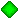Calculate magnitude formula of a newly discovered comet.

If you want to see how bright a newly discovered comet will be, you have to calculate the magnitude formula.

However, you cannot calculate the proper magnitude formula in the way mentioned in the "Light Curve Analysis of Comet Hale-Bopp" page, because the period of observations is too short soon after the discovery.

For example, the light curve of Comet Machholz, discovered in 2004 August, soon after the discovery becomes as follows.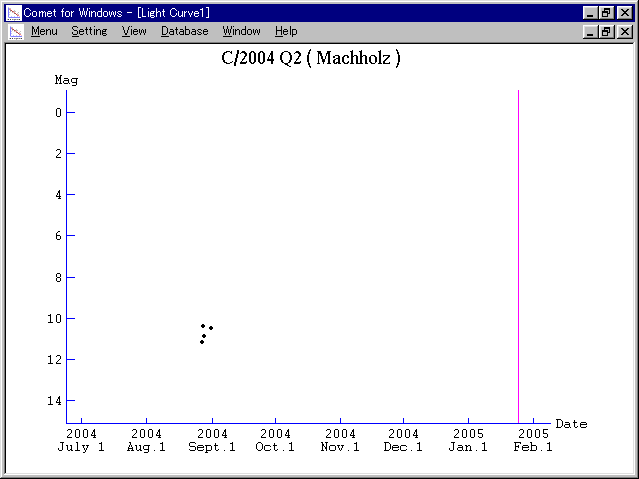Selecting "Setting" - "Calculate" menu, opening "Calculate Magnitude Formula" dialog, and calculating the formula, the following formula was displayed.

m1 = -7.77 + 5 log d + 43.28 log r

The curve of this formula becomes as follows.However, this is not correct.

When you calculate the magnitude formula with a small number of observations, you should fix the gradient, assuming the coefficient of log r as 10.

First of all, please select "Setting" - "Calculate" menu and open "Calculate Magnitude Formula" dialog. Next, please push "Calculate Magnitude Formula" button. Then the following dialog appears. Here please select "Comet (Fixed Gradient)" and input 10 in "Coefficient of log r".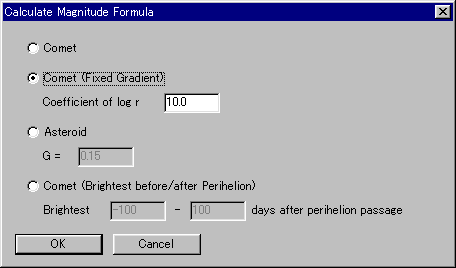After pushing OK button, the calculated magnitude formula, assuming the coefficient of log r as 10, is displayed at "Calculated Formula" part in "Calculate Magnitude Formula" dialog. In the case of this example, the following formula was calculated.

m1 = 5.20 + 5 log d + 10.0 log r

The curve of this formula becomes as follows.Now we can predict that Comet Machholz will be 4 mag at best.

##Calculate magnitude formula of an asteroid.

Some comets are very inactive and behave as if they were asteroid. In the case of those comets, you should adapt a magnitude formula as an asteroid.

For example, Comet 107P/Wilson-Harrington is also known as an asteroid 4015. The light curve of this comet becomes as follows. The green curve shows the light curve as a usual comet, but it does not coincide at all.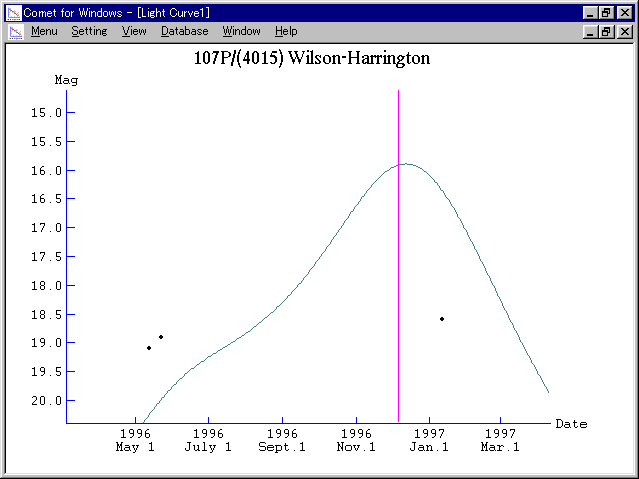So let's calculate a magnitude formula as an asteroid.

First of all, please select "Setting" - "Calculate" menu and open "Calculate Magnitude Formula" dialog. Next, please push "Calculate Magnitude Formula" button. Then the following dialog appears. Here please select "Asteroid".After pushing OK button, the calculated magnitude formula, as an asteroid, is displayed at "Calculated Formula" part in "Calculate Magnitude Formula" dialog. In the case of this example, the following formula was calculated.

H = 16.9 G = 0.15

The curve of this formula becomes as follows.The light curve coincides with the observations.

##Calculate magnitude formula of a comet whose brightest peak is shifted.

In general, a comet becomes brightest when it becomes closest to the sun. However, some periodic comets tend to become brightest some time after they become closest to the sun, when they are going away from the sun.

In order to express the light curve of those comets, there is a way to calculate the magnitude using heliocentric distance of dt days before. If a comet becomes brightest 30 days after the perihelion passage, the magnitude is calculated using heliocentric distance of 30 days before.

That magnitude formula is as follows.

m1 = m0 + 5 log delta + k log r(t - dt)

For example, Comet 6P/d'Arrest is one of those periodic comets. The light curve of this comet is as follows. The green curve shows the light curve as a usual comet, but it does not coincide at all with the observations before the perihelion passage.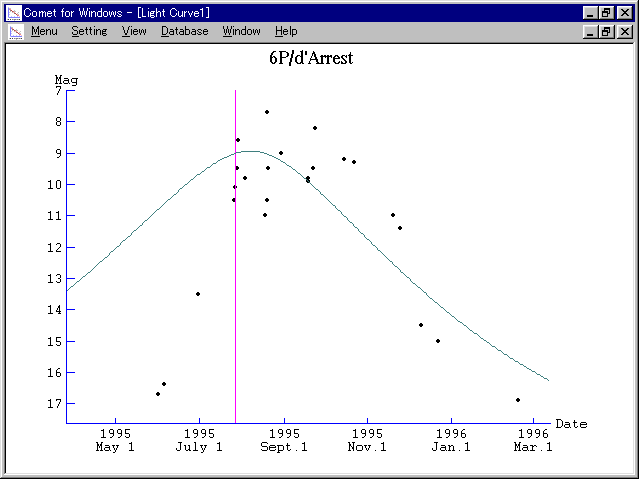So let's calculate a magnitude formula using heliocentric distance of dt days before.

First of all, please select "Setting" - "Calculate" menu and open "Calculate Magnitude Formula" dialog. Next, please push "Calculate Magnitude Formula" button. Then the following dialog appears. Here please select "Comet (Brightest before/after Perihelion)".

Please specify the range to search the time to be brightest. In this case, the comet seems to be brightest about 2 months after the perihelion passage. So please input the range of 0 days and 120 days.After pushing OK button, the calculated magnitude formula, using heliocentric distance of dt days before, is displayed at "Calculated Formula" part in "Calculate Magnitude Formula" dialog. In the case of this example, the following formula was calculated. Now we can see the comet became brightest 72 days after the perihelion passage.

m1 = 5.13 + 5 log d + 38.07 log r(t - 72)

The curve of this formula becomes as follows.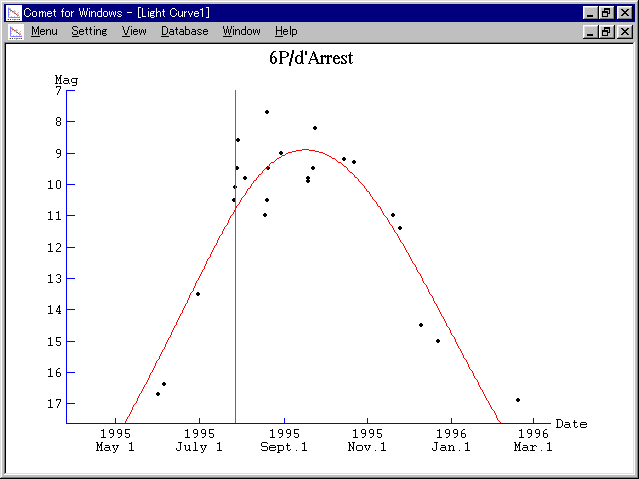The light curve coincides with the observations.